# High School Math : How to find the length of the diagonal of a hexagon

## Example Questions

### Example Question #1 : How To Find The Length Of The Diagonal Of A Hexagon

What is the length of a diagonal of a regular hexagon with side length?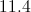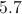Explanation:

Regular hexagons are comprised of six equilateral triangles; in our question, these triangles each have side length 4 (see diagram).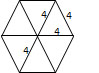The length of a diagonal is equal to two times the length of the side. In this instance, the answer is 8.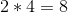### Example Question #2 : How To Find The Length Of The Diagonal Of A Hexagon

How many diagonals are there in a regular hexagon?Explanation:

A diagonal is a line segment joining two non-adjacent vertices of a polygon.  A regular hexagon has six sides and six vertices.  One vertex has three diagonals, so a hexagon would have three diagonals times six vertices, or 18 diagonals.  Divide this number by 2 to account for duplicate diagonals between two vertices. The formula for the number of vertices in a polygon is: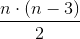where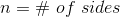.

### Example Question #3 : How To Find The Length Of The Diagonal Of A Hexagon

How many diagonals are there in a regular hexagon?

10

9

3

6

18

9

Explanation:

A diagonal connects two non-consecutive vertices of a polygon.  A hexagon has six sides.  There are 3 diagonals from a single vertex, and there are 6 vertices on a hexagon, which suggests there would be 18 diagonals in a hexagon.  However, we must divide by two as half of the diagonals are common to the same vertices. Thus there are 9 unique diagonals in a hexagon. The formula for the number of diagonals of a polygon is:where n = the number of sides in the polygon.

Thus a pentagon thas 5 diagonals.  An octagon has 20 diagonals.